Finite Mathematics : Linear Equations and Graphs

Example Questions

Example Question #1 : Linear Equations And Graphs

A pig's age, in human years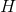, is described by the following function.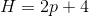In human years, how old is a four year old pig?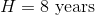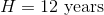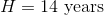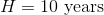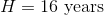Explanation:

To calculate the age of the pig in human years, first recall the equation that given in the question.Now, identify the known information given in the question.

Since the pig is four years old, the mathematical statement is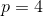Next, substitute in this value into the equation to calculate the human age.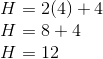Therefore, the four year old pig is twelve years old in human years.

Example Question #1 : Linear Equations And Graphs

Graph the function.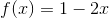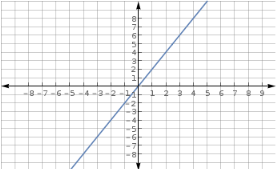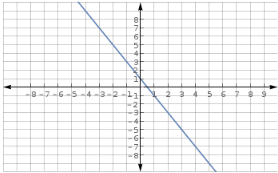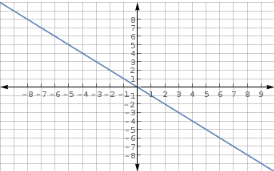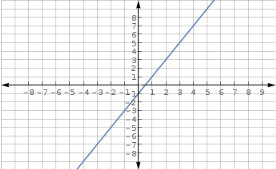Explanation:

To graph the function,

create antable to find values and then plot those values on a coordinate grid and connect the points.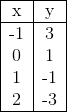Example Question #1 : Linear Equations And Graphs

Which of the following is true of the lines of the equations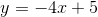and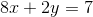The lines are parallel.

The lines intersect at one and only one point.

The lines coincide.

The lines are parallel.

Explanation:is in slope-intercept form.

To getin slope-intercept form, add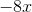to both sides, then multiply both sides by, as follows: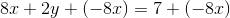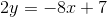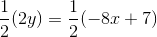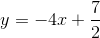The system can thus be stated asThe slope of each line is x-coefficient; the lines have different y-intercepts. This makes them distinct parallel lines.